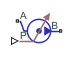# Pressure Source (IL)

Generate specified pressure differential in isothermal liquid network

• Library:
• Simscape / Foundation Library / Isothermal Liquid / Sources

•## Description

The Pressure Source (IL) block represents an ideal mechanical energy source in an isothermal liquid network. The source can maintain specified pressure differential across its ports regardless of the mass flow rate through the source. There is no flow resistance and no heat exchange with the environment.

Ports A and B represent the source inlet and outlet. The input physical signal at port P specifies the pressure differential. Alternatively, you can specify a constant pressure differential as a block parameter. A positive pressure differential causes the pressure at port B to be greater than the pressure at port A.

The block calculates the work performed on the fluid and includes the results in the simulation data log for information purposes:

`${W}_{mech}=\stackrel{˙}{m}\frac{{p}_{B}-{p}_{A}}{\overline{\rho }}$`
`$\overline{\rho }=\frac{{\rho }_{A}+{\rho }_{B}}{2}$`

where:

• Wmech is the mechanical work performed by the source.

• $\stackrel{˙}{m}$ is the mass flow rate generated by the source.

• pA and pB are pressures at ports A and B, respectively.

• $\overline{\rho }$ is the average fluid mixture density.

• ρA and ρB are fluid mixture density values at ports A and B, respectively. Equations used to compute the fluid mixture density depend on the selected isothermal liquid model. For detailed information, see Isothermal Liquid Modeling Options.

For information on viewing logged simulation data, see Data Logging.

### Assumptions and Limitations

• There are no losses due to friction.

• There is no heat exchange with the environment.

## Ports

### Input

expand all

Input physical signal that specifies the pressure differential across the source.

#### Dependencies

This port is visible if you set the Source type parameter to `Controlled`.

### Conserving

expand all

Isothermal liquid conserving port. A positive pressure differential causes the pressure at port B to be greater than the pressure at port A.

Isothermal liquid conserving port. A positive pressure differential causes the pressure at port B to be greater than the pressure at port A.

## Parameters

expand all

Select whether the pressure differential generated by the source can change during simulation:

• `Controlled` — The pressure differential is variable, controlled by an input physical signal. Selecting this option exposes the input port P.

• `Constant` — The flow rate is constant during simulation, specified by a block parameter. Selecting this option enables the Pressure differential parameter.

Desired pressure differential across the source.

#### Dependencies

Enabled when you set the Source type parameter to `Constant`.

## Version History

Introduced in R2020a